Three Phase Line Commutated Converter - 1

# Three Phase Line Commutated Converter - 1 - Notes | Study GATE Notes & Videos for Electrical Engineering - Electrical Engineering (EE)

 1 Crore+ students have signed up on EduRev. Have you?

Introduction

The three phase fully controlled bridge converter has been probably the most widely used power electronic converter in the medium to high power applications. Three phase circuits are preferable when large power is involved. The controlled rectifier can provide controllable out put dc voltage in a single unit instead of a three phase autotransformer and a diode bridge rectifier. The controlled rectifier is obtained by replacing the diodes of the uncontrolled rectifier with thyristors. Control over the output dc voltage is obtained by controlling the conduction interval of each thyristor. This method is known as phase control and converters are also called “phase controlled converters”. Since thyristors can block voltage in both directions it is possible to reverse the polarity of the output dc voltage and hence feed power back to the ac supply from the dc side. Under such condition the converter is said to be operating in the “inverting mode”. The thyristors in the converter circuit are commutated with the help of the supply voltage in the rectifying mode of operation and are known as “Line commutated converter”. The same circuit while operating in the inverter mode requires load side counter emf. for commutation and are referred to as the “Load commutated inverter”.

In phase controlled rectifiers though the output voltage can be varied continuously the load harmonic voltage increases considerably as the average value goes down. Of course the magnitude of harmonic voltage is lower in three phase converter compared to the single phase circuit. Since the frequency of the harmonic voltage is higher smaller load inductance leads to continuous conduction. Input current wave shape become rectangular and contain 5th and higher order odd harmonics. The displacement angle of the input current increases with firing angle. The frequency of the harmonic voltage and current can be increased by increasing the pulse number of the converter which can be achieved by series and parallel connection of basic 6 pulse converters. The control circuit become considerably complicated and the use of coupling transformer and / or interphase reactors become mandatory.

With the introduction of high power IGBTs the three phase bridge converter has all but been replaced by dc link voltage source converters in the medium to moderately high power range. However in very high power application (such as HV dc transmission system, cycloconverter drives, load commutated inverter synchronous motor drives, static scherbius drives etc.) the basic B phase bridge converter block is still used. In this lesson the operating principle and characteristic of this very important converter topology will be discussed in source depth.

Operating principle of 3 phase fully controlled bridge converter

A three phase fully controlled converter is obtained by replacing all the six diodes of an uncontrolled converter by six thyristors as shown in Fig. 13.1 (a)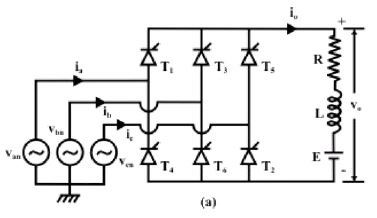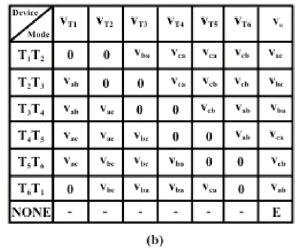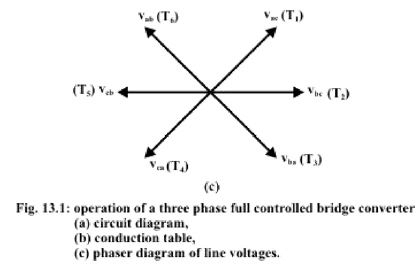For any current to flow in the load at least one device from the top group (T1, T3, T5) and one from the bottom group (T2, T4, T6) must conduct. It can be argued as in the case of an uncontrolled converter only one device from these two groups will conduct.

Then from symmetry consideration it can be argued that each thyristor conducts for 120° of the input cycle. Now the thyristors are fired in the sequence T1 → T2 → T3 → T→ T→ T6 → T1 with 60° interval between each firing. Therefore thyristors on the same phase leg are fired at an interval of 180° and hence can not conduct simultaneously. This leaves only six possible conduction mode for the converter in the continuous conduction mode of operation. These are
T1T2, T2T3, T3T4, T4T5, T5T6, T6T1. Each conduction mode is of 60° duration and appears in the sequence mentioned. The conduction table of Fig. 13.1 (b) shows voltage across different
devices and the dc output voltage for each conduction interval. The phasor diagram of the line voltages appear in Fig. 13.1 (c). Each of these line voltages can be associated with the firing of a thyristor with the help of the conduction table-1. For example the thyristor T1 is fired at the end of T5 T6 conduction interval. During this period the voltage across T1 was vac. Therefore T1 is fired α angle after the positive going zero crossing of vac. Similar observation can be made about other thyristors. The phasor diagram of Fig. 13.1 (c) also confirms that all the thyristors are fired in the correct sequence with 60° interval between each firing.

Fig. 13.2 shows the waveforms of different variables (shown in Fig. 13.1 (a)). To arrive at the waveforms it is necessary to draw the conduction diagram which shows the interval of conduction for each thyristor and can be drawn with the help of the phasor diagram of fig. 13.1
(c). If the converter firing angle is α each thyristor is fired “α” angle after the positive going zero crossing of the line voltage with which it’s firing is associated. Once the conduction diagram is drawn all other voltage waveforms can be drawn from the line voltage waveforms and from the conduction table of fig. 13.1 (b). Similarly line currents can be drawn from the output current and the conduction diagram. It is clear from the waveforms that output voltage and current waveforms are periodic over one sixth of the input cycle. Therefore this converter is also called the “six pulse” converter. The input current on the other hand contains only odds harmonics of the input frequency other than the triplex (3rd, 9th etc.) harmonics. The next section will analyze the operation of this converter in more details.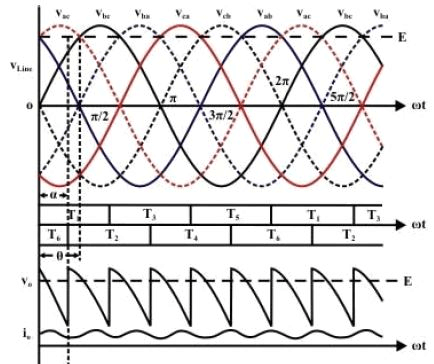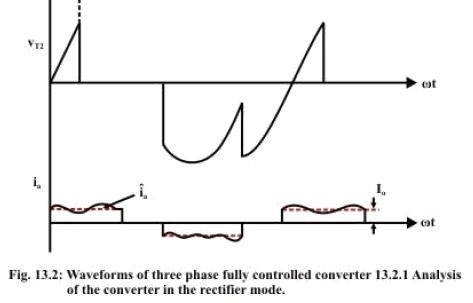Analysis of the converter in the rectifier mode

The output voltage waveform can be written as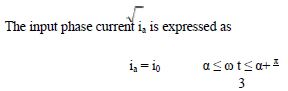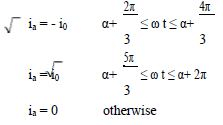From Fig. 13.2 it can be observed that i0 itself has a ripple at a frequency six times the input frequency. The closed from expression of i0, as will be seen later is some what complicated. However, considerable simplification in the expression of ia can be obtained if i0 is replaced by its average value I0. This approximation will be valid provided the ripple on i0 is small, i.e, the load is highly inductive. The modified input current waveform will then be ia which can be expressed in terms of a fourier series as
To find out the condition for continuous conduction it is noted that in the limiting case of continuous conduction.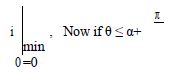then i0 is minimum at ωt = α. ∴ Condition

for continuous conduction is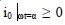. However discontinuous conduction is rare in these conversions and will not be discussed any further.

Analysis of the converter in the inverting mode.

In all the analysis presented so far it has been assumed that α < 90°. It follows from equation 13.2 that the output dc voltage will be positive in this case and power will be flowing from the three phase ac side to the dc side. This is the rectifier mode of operation of the converter. However if α is made larger than 90° the direction of power flow through the converter will reverse provided there exists a power source in the dc side of suitable polarity. The converter in that case is said to be operating in the inverter mode. It has been explained in connection with single phase converters that the polarity of EMF source on the dc side [Fig. 13.1(a)] would have to be reversed for inverter mode of operator. Fig. 13.3 shows the circuit connection and wave forms in the inverting mode of operation where the load current has been assumed to be continuous and ripple free.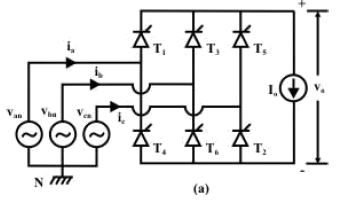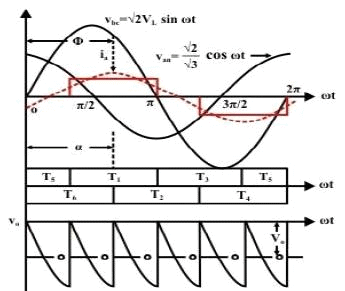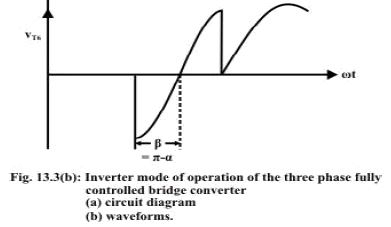Analysis of the converter in the inverting mode is similar to its rectifier mode of operation. The same expressions hold for the dc and harmonic compounds in the output voltage and current. The input supply current Fourier series is also identical to Equation 13.8. In particular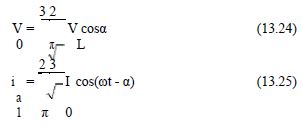For values of α in the range 90° < α < 180° it is observed from Fig. 13.3(b) that the average dc voltage is negative and the displacement angle φ of the fundamental component of the input ac line current is equal to α > 90°. Therefore, power in the ac side flows from the converter to the source.

It is observed form Fig. 13.3(b) that an outgoing thyristor (thyristor Tin Fig. 13.3(b)) after commutation is impressed with a negative voltage of duration β = π – α. For successful commutation of the outgoing thyristor it is essential that this interval is larger than the turn off time of the thyristor i.e,

β ≥ ωtq , tq is the thyristor turn off time

Therefore π- α ≥ ωtq or α ≤ π- ωtq .

Which imposes an upper limit on the value of α. In practice this upper value of α is further reduced due to commutation overlap.

Exercise 13.2

A three phase fully controlled bridge converter operating from a 3 phase 220 V, 50 Hz supply is used to charge a battery bank with nominal voltage of 240 V. The battery bank has an internal resistance of 0.01 Ω and the battery bank voltage varies by ± 10% around its nominal value between fully charged and uncharged condition. Assuming continuous conduction find out.
- The range of firing angle of the converter.
- The range of ac input power factor.
- The range of charging efficiency.

When the battery bank is charged with a constant average charging current of 100 Amps through a 250 mH lossless inductor.

Answer: The maximum and minimum battery voltages are, VB Min = 0.9 × VB Nom = 216 volts and VB Max = 1.1 × VB Nom = 264 volts respectively.

Since the average charging current is constant at 100 A.

V0 Max = VB Max + 100 × RB = 264 + 100 × 0.01 = 265 volts
V0 Min = VB Min + 100 × R= 216 + 100 × 0.01 = 217 volts.

2. A three phase fully controlled converter operates from a 3 phase 230 V, 50 Hz supply through a Y/ transformer to supply a 220 V, 600 rpm, 500 A separately excited dc motor. The motor has an armature resistance of 0.02 Ω. What should be the transformer turns ratio such that the converter produces rated motor terminal voltage at 0º firing angle. Assume continuous conduction. The same converter is now used to brake the motor regeneratively in the reverse direction. If the thyristors are to be provided with a minimum turn off time of 100 μs, what is the maximum reverse speed at which rated braking torque can be produced.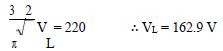Where VL is the secondary side line and also the phase voltage since the secondary
side is connected.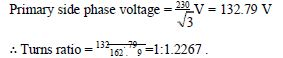During regenerative braking in the reverse direction the converter operates in the inverting mode.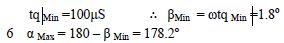7 Maximum negative voltage that can be generated by the converter is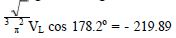V For rated braking torque Ia = 500 A
∴ Eb = Va – Iara = - 229.89 V.
At 600 RPM Eb = 220 – 500 × 0.02 = 210 V.

∴ Max reverse speed is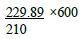= 656.83 RPM .

The document Three Phase Line Commutated Converter - 1 - Notes | Study GATE Notes & Videos for Electrical Engineering - Electrical Engineering (EE) is a part of the Electrical Engineering (EE) Course GATE Notes & Videos for Electrical Engineering.
All you need of Electrical Engineering (EE) at this link: Electrical Engineering (EE)

## GATE Notes & Videos for Electrical Engineering

28 videos|342 docs
 Use Code STAYHOME200 and get INR 200 additional OFF

## GATE Notes & Videos for Electrical Engineering

28 videos|342 docs

Track your progress, build streaks, highlight & save important lessons and more!

,

,

,

,

,

,

,

,

,

,

,

,

,

,

,

,

,

,

,

,

,

;